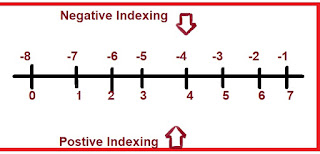## Data Types In Python

this post is all about the data types in python explained with examples and images for a better understanding of this concept.

When we are working on any project, It's very important to understand what type of data you are working with so that you can process that data in an appropriate format. If you don't process data in an inappropriate format you might face errors and bugs.

### What are the different Data Types in PythonDATA TYPES IN PYTHON
The above diagram shows the different data types in python but each data type have different types and some integral data types lets see one by one below this.

### NONE Data type in python

When you have a variable and that variable is not assigned with any value that is called NONE. in different languages we use NULL but in Python we use NONE. this data type defines that the variable doesn't hold any value as of now.but later you can change the value in None Variable.
• None is not 0
• It's not an empty string at all
• None doesn't represent False

SYNTAX: NONE

Examples

>>> a =None

>>> print("",a);
None
>>> print("The value of a is",a);
The value of a is None

### Numeric Data type in Python

As its name suggests,This is the data type which stored numbers and we know there are different types of numbers like natural, complex, decimal, etc.

Similarly, Python have different data types in the Numeric Data type Section.Numeric Data type

#### int Data type in Python

an int data type is deal with the whole numbers(0,1,2,3,4, etc), these numbers can be positive or negative, also numbers don't have any decimal points.

In C language size of int is 4 bytes but in python3 the size of int is (24 bytes) unlimited and in python2 we can use long with int to increase the size of the data type.

>>> num =13
>>> num2=14
>>> num3=16
>>> num+num2+num3
43

#### float Data type in Python

Float values are number with decimal points, like C and C++ we don't have to write the data type in python we can directly write the number without mentioning its data type.

>>> a=14
>>> b=16
>>> c=1.412
>>> d=19.65
>>> a+b+c+d
51.062

As you can see from above the answer, it has decimal point and we did not mention float data type anywhere in the above code and still get the answer as a float value

#### Complex Data type in Python

First, we need to know, What is a complex number?
Complex number is written in the form a+ib where "a" is called the real part which is (1,2,3,4,5, etc) and "ib" is called the imaginary number (i=under root -1).
But, In python, we use "j" instead of "i".
complex data type typically used in mathematical functions.

>>>14+9j
>>>19+4j

#### Boolean Data type in Python

Boolean data type is have two options either it will be true or false, in python we define boolean with True or False keywords.
"Remember that the intial letter in True and False in booloean Data type must be upper Case or the compiler will give error"
>>> a=4
>>> b=9
>>> b>a
True
>>> a>b
False
>>> bool =a>b  Here we have assigned the Boolean to the bool and we give one condition to that variable and when we press enter the value in bool will be either True or False
>>> bool
False  As you can see here the value in bool is false as per our condition.
>>> a+8>b
True
>>> a+3<b
True
>>> a+9<b
False

"The common boolean operators are given below"

• and
• not
• or
• ==(Equal to)
• !=(Not equal to)

>>> X=True
>>> Y=False
>>> X or Y
True
>>> Y or X
True
>>> Y and X
False
>>> X==Y
False
>>> X!=Y

True
>>> not X
False
>>> not Y
True

 X Y X==Y X or Y X and Y True False False True False False True False True False True True True True True False False True False False

True posses value 1
False posses value 0

### List in Python

List is same as array(the array is the collection of data of same data type) but list not necessarily of homogeneous data type that why this quality makes it one of the most powerful tool in python language.

#### Some facts about list

• we can use integer, float, string, etc data type in one list.
• List are mutable in nature ,Hence we can change values as per requirement.
• List is Ordered
• List have Definite count
• List uses concept of Index number(https://www.digitalzak.com/2020/01/pythonlistappend.html) To know what is index number you can visit this link.
• Duplication of the element in the list is allowed because index numbers are fixed for each element.indexing in python

Example

>>> list=[12,13,14,15,18,12,12,16]
>>> print(list)
15
>>> list
[12, 13, 14, 15, 18, 12, 12, 16]
>>> digitalzak=["Zeeshan","MBA","Data","Python"]
>>> print(digitalzak)
Data
>>> print(digitalzak)
Zeeshan

We Can add values and remove values from the list by using certain functions and to know more about it you can visit the link.

### Tuple in Python Link this is link

Visit this link for Tuple with example and overview.

### Range in Python

The range function is used to show the range of starting to the end of the series, for example, range from 1-6
then it will show 1,2,3,4,5,6 this numbers.

By using LIST function we can print the entire range.

"Thanks for reading for more post on python you can follow my blog so that you will notify about new post"